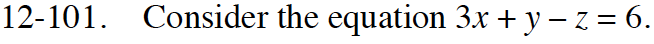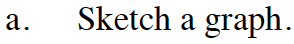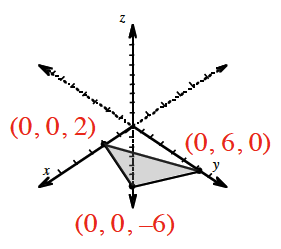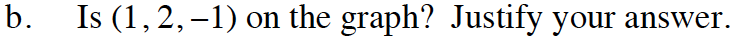### Home > INT3 > Chapter Ch12 > Lesson 12.2.2 > Problem12-101

12-101.
1. Consider the equation 3x + yz = 6. Homework Help ✎

1. Sketch a graph.

2. Is (1, 2, –1) on the graph? Justify your answer.Notice that there are three variables. Find the three intercepts.Does the point make the equation true?

Yes, it is a solution to the equation.